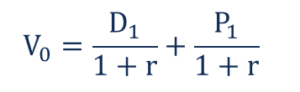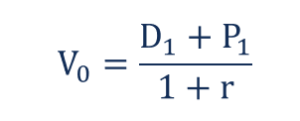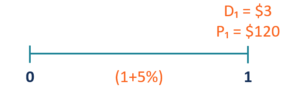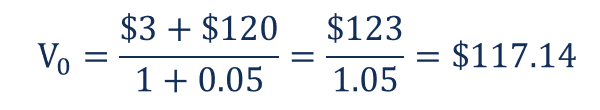# One-Period Dividend Discount Model

How to calculate the intrinsic value of a stock that is planned to be held for one period only

## What is the One-Period Dividend Discount Model (DDM)?

The one-period dividend discount model is a variation of the dividend discount model. The one-period dividend discount variation is used to determine the intrinsic value of a stock that is planned to be held for one period only (usually one year).

Similar to the general dividend discount model, the one-period variation is based on the assumption that the intrinsic value of a stock equals the sum of all future cash flows discounted back to its present value.In such a case, when an investor is willing to hold the stock only for one year, there are only two possible cash flows that are generated by the stock: one dividend payment and the selling price of the stock. Thus, in order to find the current fair price of a stock, we must calculate the sum of the future dividend payment and the anticipated selling price discounted back to their present values.

### Formula for the One-Period Dividend Discount Model

The mathematical formula that allows calculating the intrinsic value of a stock using the one-period dividend discount model is given below:Where:

• V– the current fair value of a stock
• D– the dividend payment in one period from now
• P– the stock price in one period from now
• r – the estimated cost of equity capital

The formula above can be simplified into the following manner:### Example of the One-Period Dividend Discount Model

Assume that you are an investment analyst. Your client has asked you to assess the viability of their investment in ABC Corp. The client expects to hold the investment for one year only and sell it at the end of the holding period.

You’ve discovered that ABC Corp. will pay \$3 in dividends per share, while the selling price at the end of the holding period can reach \$120 per share. The estimated cost of equity capital is 5%. Currently, the stock of ABC Corp. trades at \$118 per share.In order to assess the viability of the investment, you should determine the intrinsic value of the company’s stock. It can be found using the one-period dividend discount model. By inputting the known variables into the formula above, the intrinsic stock value can be calculated in the following way:The intrinsic value of the company’s stock is \$117.14, which is less than the company’s current stock price (\$118). Therefore, we can say that the stock is currently overvalued.

Thank you for reading CFI’s explanation of the one-period dividend discount model. CFI is the official provider of the Financial Modeling and Valuation Analyst (FMVA)™ certification program, designed to transform anyone into a world-class financial analyst.

To keep learning and developing your knowledge of financial analysis, we highly recommend the additional resources below:

• Dividend Growth Rate
• Ex-Dividend Date
• FCFF vs FCFE vs Dividends
• Multiple-Period Dividend Discount Model

### Financial Analyst Training

Get world-class financial training with CFI’s online certified financial analyst training program!

Gain the confidence you need to move up the ladder in a high powered corporate finance career path.

Learn financial modeling and valuation in Excel the easy way, with step-by-step training.